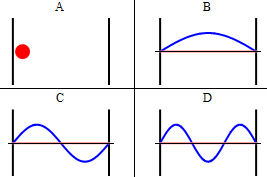Lecture 9: More on PIB and Orthonormality

•• Contributed by Delmar Larsen
• Founder and Director at Libretexts

Recap of Lecture 8:

Last lecture focused on gaining an intuition of wavefunctions with an emphasis on the particle in the box. Specifically, we considered the four principal properties of continuous distributions given in Worksheet 1 and applied it to the particle in the box. This must be mastered for the exam. We want to develop an intuition behind how the energy and wavefunctions change in PIB when mass is increased, when box length is increased and when quantum number n is increased.

We ended the discussion in class focusing on a powerful (blow your mind) fact that the eigenstates of an operator will be orthoganal.

$\int_{0}^{L}\psi _{m}(x)\psi _{n}(x)dx=0\: \: \: if\: \: \: m \neq n \label{3.5.19}$

This is really cool as we will see. So, ideal we want wavefunctions to be normalized and they are intrinsically orthoganal (from solving the eigenvalue/vector problem).

Full Time Dependence

Recall that the time-dependence of the wavefucntion with time-independent potential was discussed in Section 3.1 and is expressed as

$\Psi(x,t)=\psi(x)e^{-iEt / \hbar}$

so for the particle in a box, these are

$\psi _{n}(x)= \underbrace{\sqrt{\dfrac{2}{L}} \sin \dfrac{n\pi x}{L}}_{\text{spatial part}} \overbrace{e^{-iE_nt / \hbar}}^{\text{temporal part}} \label{PIBtime}$

with $$E_n$$ given by

$E_{n}=\dfrac{\hbar^2\pi^2}{2mL^2}\, n^2=\dfrac{h^2}{8mL^2}n^2 \label{3.5.11}$

with $$n=1,2,,3...$$.

The phase part of Equation \ref{PIBtime} can be expanded into a real part and a complex components. So the total wavefunction for a particle in a box is

$\Psi(x,t)= \underbrace{\left(\sqrt{\dfrac{2}{L}} \sin \dfrac{n\pi x}{L}\right) \left(\cos \dfrac{E_nt}{\hbar}\right)}_{\text{real part}} - \underbrace{i \left(\, \sqrt{\dfrac{2}{L}} \sin \dfrac{n\pi x}{L} \right) \left(\sin \dfrac{E_nt}{\hbar} \right) }_{\text{imaginary part}}$

which can be simplified (slightly) to

$\Psi(x,t)= \underbrace{\left(\sqrt{\dfrac{2}{L}} \sin \dfrac{n\pi x}{L}\right) \left(\cos \dfrac{E_nt}{\hbar}\right)}_{\text{real part}} - \underbrace{i \left(\, \sqrt{\dfrac{2}{L}} \sin \dfrac{n\pi x}{L} \right) \left(\cos \dfrac{E_nt}{\hbar} - \dfrac{\pi}{2} \right) }_{\text{imaginary part}}$

As discussed previously, the imaginary part of the total wavefunction oscillates out of phase by $$π/2$$ with respect to the real part (we call this "out of phase"). This is demonstrated in the time-dependent behavior of the first three eigenfunctions below.Some trajectories of a particle in a box according to Newton's laws of classical mechanics (A), and according to the Schrödinger equation of quantum mechanics (B-F). In (B-D), the horizontal axis is position, and the vertical axis is the real part (blue) and imaginary part (red) of the wavefunction. The states (B,C,D) are energy eigenstates.

Java simulation of particles in boxes :https://phet.colorado.edu/en/simulation/bound-states

on board

Orthonormality

Another important property of the eigenfunctions applies to the integral over a product of two different eigenfunctions. It is easy to see from Figure $$\PageIndex{5}$$ that the integral

$\int_{0}^{a}\psi _{2}(x)\psi _{1}(x)dx=0 \label{3.5.18}$Figure $$\PageIndex{5}$$: Product of n=1 and n=2 eigenfunctions

To prove this result in general, use the trigonometric identity

$\sin\,\alpha \: \sin\, \beta =\dfrac{1}{2}\begin{bmatrix}cos(\alpha -\beta )-\cos(\alpha +\beta )\end{bmatrix}$

to show that

$\int_{0}^{L}\psi _{m}(x)\psi _{n}(x)dx=0$

if $$m \neq n$$.

This property is called orthogonality​. We will show in the next Chapter, that this is a general result from quantum-mechanical eigenfunctions. The normalization (Equation $$\ref{3.5.18}$$) together with the orthogonality (Equation $$\ref{3.5.19}$$) can be combined into a single relationship

$\int_{0}^{L}\psi _{m}(x)\psi _{n}(x)dx=\delta _{mn}\label{3.5.20}$

In terms of the Kronecker delta

$\delta _{mn}\equiv \begin{bmatrix}1\; if\; m=n\\ 0\; if\; m\neq n\end{bmatrix}\label{3.5.21}​$

A set of functions $$\begin{Bmatrix}\psi_{n}\end{Bmatrix}$$ which obeys Equation $$\ref{3.5.20}$$ is called orthonormal.